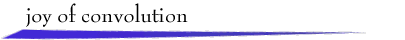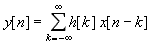The behavior of a linear, time-invariant discrete-time system with input signal x[n] and output signal y[n] is described by the convolution sumThe signal h[n], assumed known, is the response of the system to a unit-pulse input.

The convolution summation has a simple graphical interpretation. First, plot h[k] and the "flipped and shifted" x[n - k] on the k axis, where n is fixed. Second, multiply the two signals to obtain a plot of the summand sequence indexed by k. Summing the values of this sequence with respect to k yields y[n]. These operations can be repeated for every value of n of interest.

To explore graphical convolution, select signals x[n] and h[n] from the provided examples below, or use the mouse to draw your own signals or to modify selected signals. Then click at a desired value of n on the first k axis. After a moment, h[k] and x[n - k] will appear. Drag the n symbol along the k axis to change the value of n. For each n, the corresponding summand h[k]x[n - k] and output value y[n] will be displayed in their respective windows.return to demonstrations page

 Continuous-time version. Original applet by Steve Crutchfield. Update by Michael Ross.